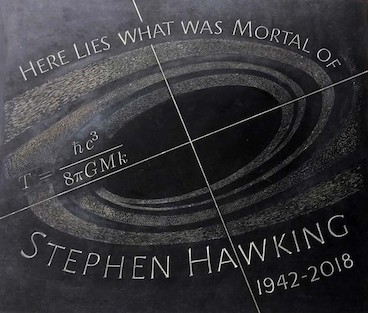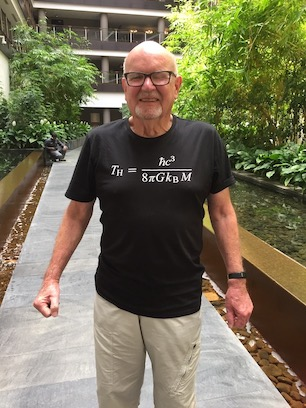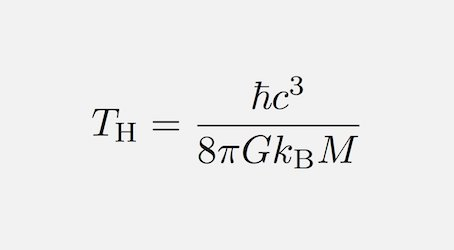Stephen Hawkings Epitaph
Stephen Hawking's Epitaph
His formula for the temperature of a Black Hole

by Tony Heyes

Stephen Hawking's formula for the temperature of a Black Hole is inscribed on his tombstone in Westminster Abbey and also on one of my tee shirts. My tribute to an old friend.

Stephen was never afraid of an arument and never shy of a controvesy. When in 1972 Jacob Bekenstein suggested that there should be thermodynamics of black holes Stephen was one of his detractors. Subsequent anslysis led to a change of mind after which Stephen persued the subject with enthusiasm.

Stepehen's temperature formula is most remarkable. It is the only formula I know which incorporates both Classical Constants and Quantum Constants.

Actually, Hawking's somewhat similar formula for the Entropy of a Black Hole also does this.
I had always understood that it was this latter formula that Stephen wished to have as his epitaph!For more information as to the significance of this formula listen to my radio interview

On Stephen Hawking's Epitaph

Those, like me, who enjoy doing calculations will need to know the values of the various constants; so here they are:

c  Velocity of light  =  299,792,458  m s-1

h bar   Planck's concstant/2pi    =  1.05 x 10^-34  J s

pi   =   3.14

G  Newton's Gravitational constant    =  6.67 x 10^-11  m3 kg-1 s-2

kb  Boltzmann's constant    =  1.38 x 10^-23  m2 kg s-2 K-1

Given that the above are all constants the temperature equation may be reduced to:

T  =  (6 x 10^-8)/Ms deg K

where Ms = the number of solar masses.

Note: the mass of the sun  =   1.989 X 10^30  kg
the mass of the moon  =  7.347 X 10^22  kg
the Cosmic Microwave Background radiation temperature =  2.725  deg K
the diameter of a Black Hole  =   2 X the Schwarzschild radius  =  4GM/c^2 m

Now it is easy to work out the mass of tbe Black Hole whose temperature equals that of tbe Cosmic Background Radiation.

Notice that it is approximately equal tp the mass of our Moon and has the diameter of a human hair!

These web pages have been written by Tony Heyes
of Perceptual Alternatives  usingArachnophilia.
andIrfanView.
They have been tested using the ubiquitous Internet Explorer
and also with my browser of choiceMozilla FireFox.